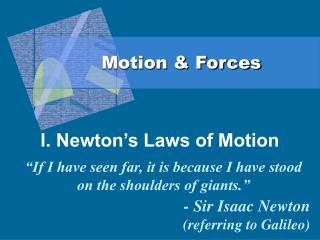# Motion & Forces - PowerPoint PPT PresentationDownload PresentationMotion & Forces

Motion & ForcesDownload Presentation## Motion & Forces

- - - - - - - - - - - - - - - - - - - - - - - - - - - E N D - - - - - - - - - - - - - - - - - - - - - - - - - - -
##### Presentation Transcript

1. Motion & Forces I. Newton’s Laws of Motion • “If I have seen far, it is because I have stood on the shoulders of giants.” • - Sir Isaac Newton • (referring to Galileo)

2. A. Newton’s First Law • Newton’s First Law of Motion • An object at rest will remain at rest and an object in motion will continue moving at a constant velocity unless acted upon by a net force.

3. B. Newton’s Second Law • Newton’s Second Law of Motion • The acceleration of an object is directly proportional to the net force acting on it and inversely proportional to its mass. F = ma a = F/m

4. C. Newton’s Third Law • Newton’s Third Law of Motion • When one object exerts a force on a second object, the second object exerts an equal but opposite force on the first.

5. STRUCTURE CHALLENGE! • Your challenge is to make a structure that catapults a marshmallow that can hit the bull's-eye